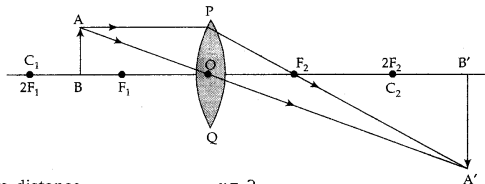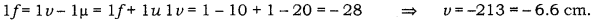# “A convex lens can form a magnified erect as well as magnified inverted image of an object placed in' front of it.”

“A convex lens can form a magnified erect as well as magnified inverted image of an object placed in’ front of it.” Draw ray diagram to justify this statement stating the position of the object with respect to the lens in each case.
An object of height 4 cm is placed at a distance of 20 cm from a concave lens of focal length 10 cm. Use lens formula to determine the position of the image formed.

(a) Magnified erect image (When the object is placed between O and F1)(b) Magnified inverted image (When the object is placed between F1 and 2F1
Object distance, u= 20 cmImage distance, v= ?
Focal length, f = 10 cm
As per the sign conventions used,
f = -10
u = -20 cm
According to the lens formula,The image is formed at a distance of 6.6 cm from the lens at the same side where the object is placed.